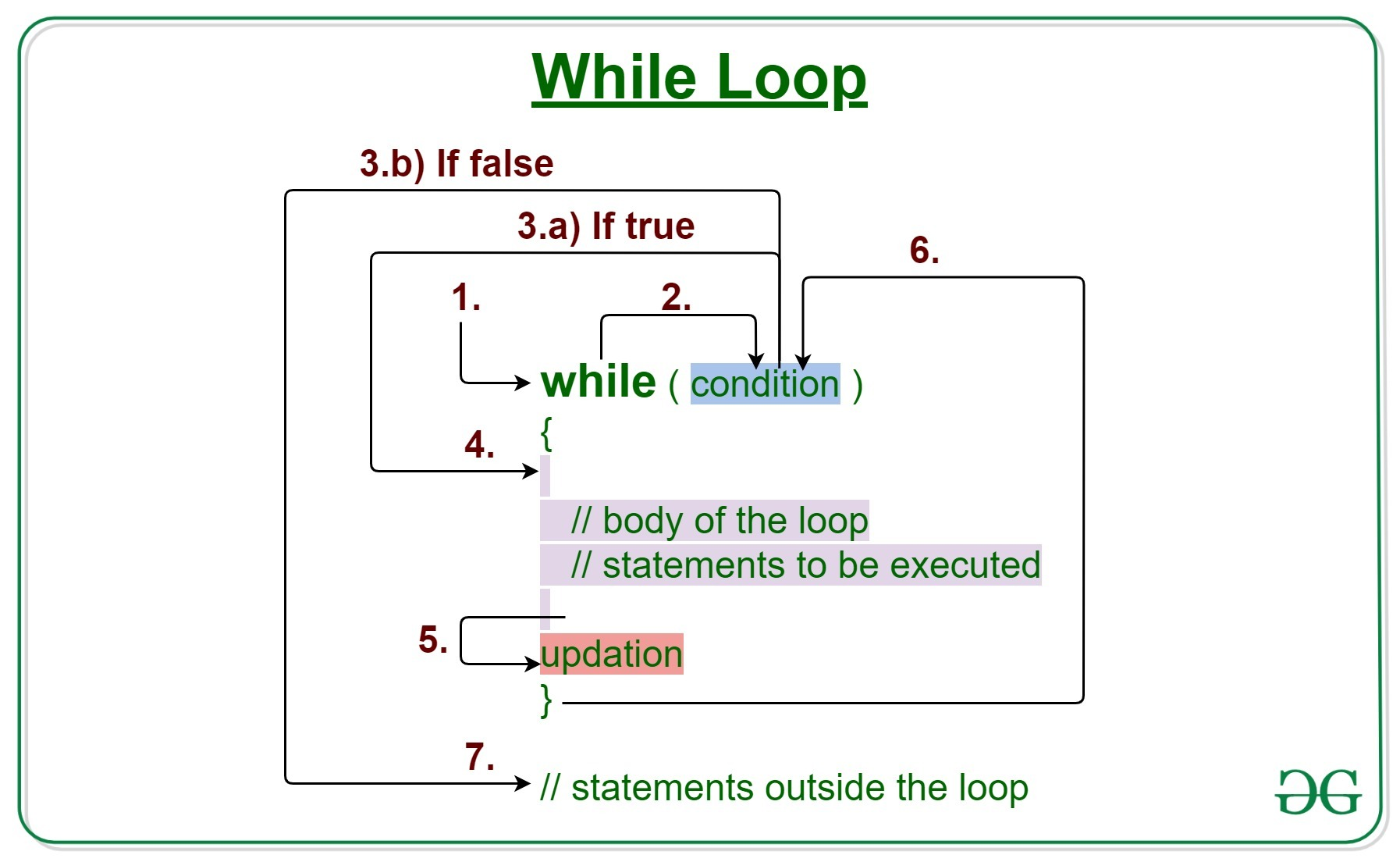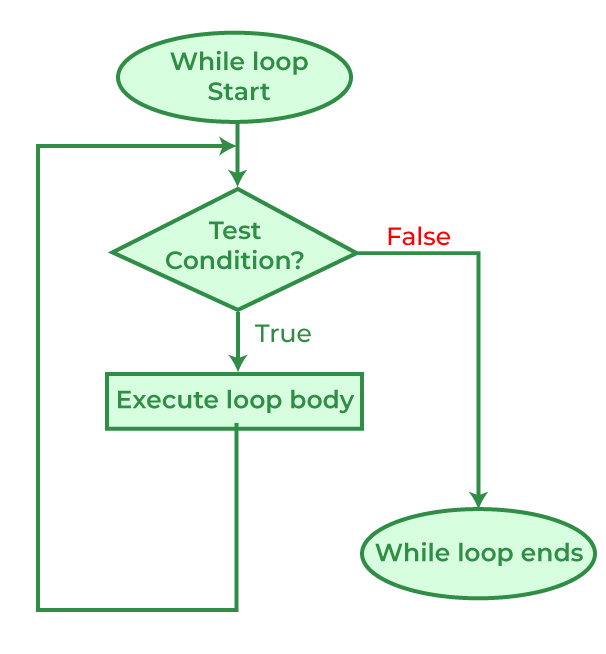Open In App
Related Articles
• Write an Interview Experience
• C++ Programming Language

# C++ While Loop

While Loop in C++ is used in situations where we do not know the exact number of iterations of the loop beforehand. The loop execution is terminated on the basis of the test condition. Loops in C++ come into use when we need to repeatedly execute a block of statements. During the study of the ‘for’ loop in C++, we have seen that the number of iterations is known beforehand, i.e. the number of times the loop body is needed to be executed is known to us.Syntax:

```while (test_expression)
{
// statements

update_expression;
}```

The various parts of the While loop are:

1. Test Expression: In this expression, we have to test the condition. If the condition evaluates to true then we will execute the body of the loop and go to update expression. Otherwise, we will exit from the while loop.
2. Update Expression: After executing the loop body, this expression increments/decrements the loop variable by some value.
3. Body: This is a group of statements that include variables, functions, and so on. With the while loop, code, and simple names can be printed, complex algorithms can be executed, or functional operations can be performed.

## How does a While loop execute?

1. Control falls into the while loop.
2. The flow jumps to Condition
3. Condition is tested.
• If the Condition yields true, the flow goes into the Body.
• If the Condition yields false, the flow goes outside the loop
4. The statements inside the body of the loop get executed.
5. Updation takes place.
6. Control flows back to Step 2.
7. The while loop has ended and the flow has gone outside.

## Flow Diagram of while loopExample 1: This program will try to print “Hello World” 5 times depending on a few conditions.

## C++

 `// C++ program to illustrate while loop`` ` `#include ``using` `namespace` `std;`` ` `int` `main()``{``    ``// initialization expression``    ``int` `i = 1;`` ` `    ``// test expression``    ``while` `(i < 6) {``        ``cout << ``"Hello World\n"``;`` ` `        ``// update expression``        ``i++;``    ``}`` ` `    ``return` `0;``}`

Output:

```Hello World
Hello World
Hello World
Hello World
Hello World```

Dry-Run of Example 1:

```1. Program starts.
2. i is initialized with value 1.
3. Condition is checked. 1 < 6 yields true.
3.a) "Hello World" gets printed 1st time.
3.b) Updation is done. Now i = 2.
4. Condition is checked. 2 < 6 yields true.
4.a) "Hello World" gets printed 2nd time.
4.b) Updation is done. Now i = 3.
5. Condition is checked. 3 < 6 yields true.
5.a) "Hello World" gets printed 3rd time
5.b) Updation is done. Now i = 4.
6. Condition is checked. 4 < 6 yields true.
6.a) "Hello World" gets printed 4th time
6.b) Updation is done. Now i = 5.
7. Condition is checked. 5 < 6 yields true.
7.a) "Hello World" gets printed 5th time
7.b) Updation is done. Now i = 6.
8. Condition is checked. 6 < 6 yields false.
9. Flow goes outside the loop to return 0.```

Example 2:

## C++

 `// C++ program to illustrate while loop`` ` `#include ``using` `namespace` `std;`` ` `int` `main()``{``    ``// initialization expression``    ``int` `i = 1;`` ` `    ``// test expression``    ``while` `(i > -5) {``        ``cout << i << ``"\n"``;`` ` `        ``// update expression``        ``i--;``    ``}`` ` `    ``return` `0;``}`

Output:

```1
0
-1
-2
-3
-4```